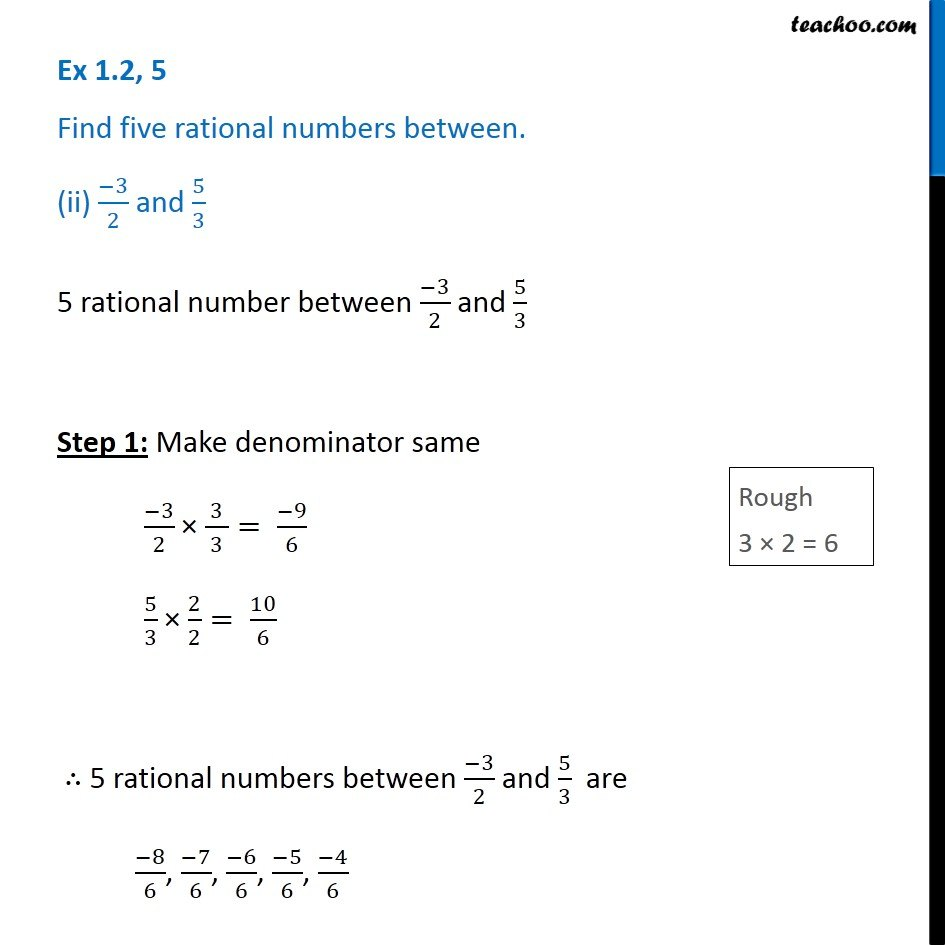Ex 1.2

Chapter 1 Class 8 Rational Numbers
Serial order wiseGet live Maths 1-on-1 Classs - Class 6 to 12

### Transcript

Ex 1.2, 5 Find five rational numbers between. (ii) (−3)/2 and 5/3 5 rational number between (−3)/2 and 5/3 Step 1: Make denominator same (−3)/2 × ( 3 )/3= (−9)/6 5/3 × 2/2= 10/6 ∴ 5 rational numbers between (−3)/2 and 5/3 are (−8)/6, (−7)/6, (−6)/6, (−5)/6, (−4)/6